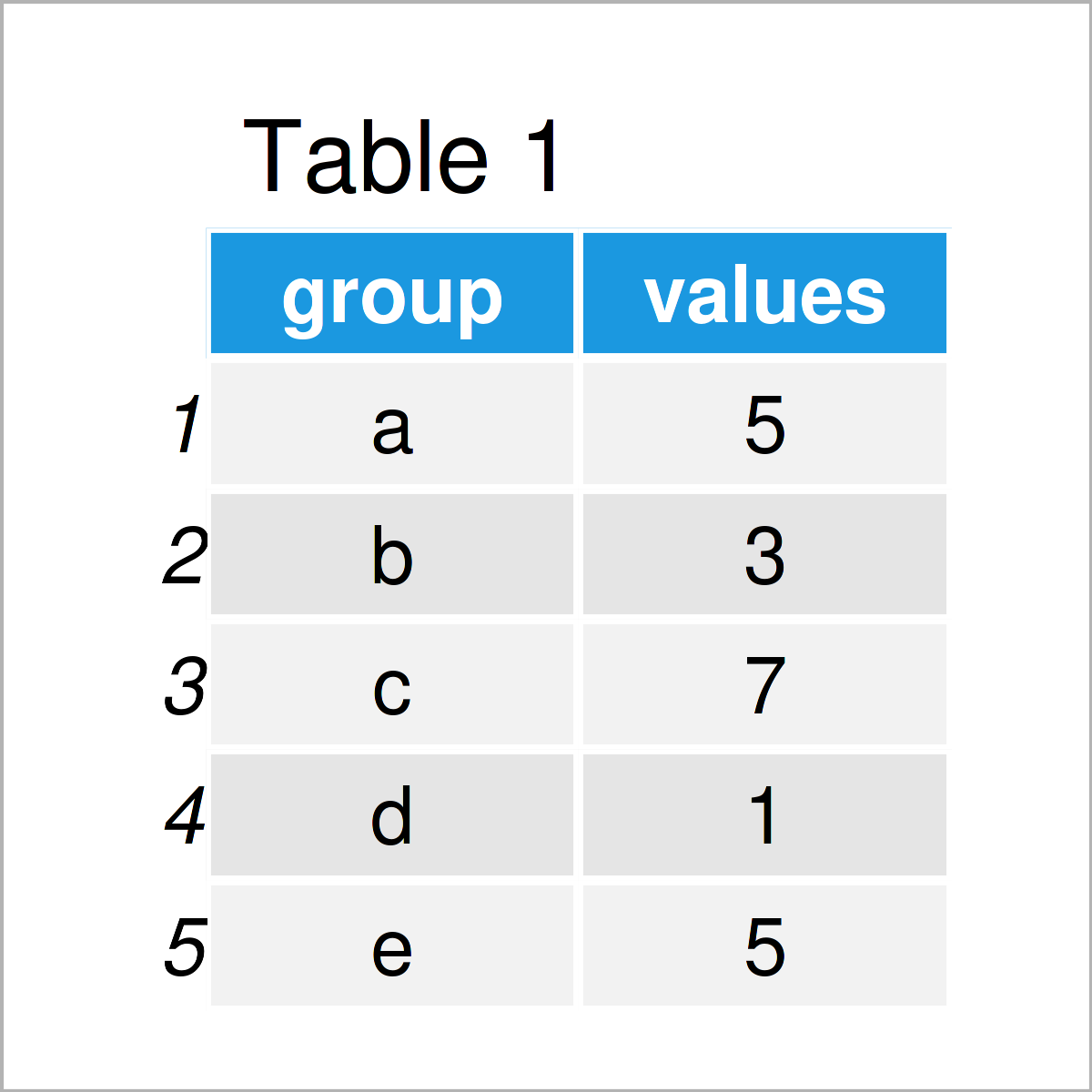# R ggplot2 Error: StatBin requires a continuous x variable: the x variable is discrete. Perhaps you want stat=”count”?

This article demonstrates how to handle the “Error: StatBin requires a continuous x variable: the x variable is discrete.Perhaps you want stat=”count”?” in the R programming language.

The tutorial contains the following information:

Let’s dig in…

## Example Data & Packages

I’ll use the following data as basement for this R tutorial:

```data <- data.frame(group = letters[1:5],      # Create example data
values = c(5, 3, 7, 1, 5))
data                                          # Print example data```Table 1 shows that our exemplifying data contains five rows and two variables. The variable group has the character class and the variable values has the numeric class.

In order to plot our data with the ggplot2 package, we also need to install and load ggplot2:

```install.packages("ggplot2")                   # Install ggplot2 package

So far, so good – Let’s move on to the example codes!

## Example 1: Reproduce the Error: StatBin requires a continuous x variable

In this example, I’ll show how to replicate the “Error: StatBin requires a continuous x variable: the x variable is discrete.Perhaps you want stat=”count”?”.

Suppose we want to draw our data in a graphic with bars. Then, we might try to create a ggplot2 histogram using the following R code:

```ggplot(data, aes(x = group)) +                # geom_histogram does not work
geom_histogram()
# Error: StatBin requires a continuous x variable: the x variable is discrete.Perhaps you want stat="count"?```

Unfortunately, the RStudio console returns the “Error: StatBin requires a continuous x variable: the x variable is discrete.Perhaps you want stat=”count”?”.

The reason for this is that we cannot draw a histogram of a character of factor variable. Histograms are only suited for numerical data.

So how could we draw our data properly?

## Example 2: Fix the Error: StatBin requires a continuous x variable

This example explains how to avoid the “Error: StatBin requires a continuous x variable: the x variable is discrete.Perhaps you want stat=”count”?”.

To do this, we could draw a barchart of our data instead of a histogram. Have a look at the following R code:

```ggplot(data, aes(x = group, y = values)) +    # geom_bar works fine
geom_bar(stat = "identity")```As shown in Figure 1, the previous R programming syntax has plotted a ggplot2 plot of our data without showing any error messages.

## Video & Further Resources

Would you like to know more about the ggplot2 package? Then you may have a look at the following video of my YouTube channel. I explain how to use the ggplot2 package in much more detail:

Please accept YouTube cookies to play this video. By accepting you will be accessing content from YouTube, a service provided by an external third party.If you accept this notice, your choice will be saved and the page will refresh.

Furthermore, you might want to read the related articles on this website. I have published several tutorials already:

In summary: This tutorial has illustrated how to deal with the “Error: StatBin requires a continuous x variable: the x variable is discrete.Perhaps you want stat=”count”?” in the R programming language. Let me know in the comments, if you have further comments or questions.

Subscribe to the Statistics Globe Newsletter